$\def\D{\Omega} \def\ipar{m} \def\R{\mathbb{R}} \def\del{\partial} \def\vec{\bf} \def\priorm{\mu_0} \def\C{\mathcal{C}} \def\Acal{\mathcal{A}} \def\postm{\mu_{\rm{post}}} \def\iparpost{\ipar_\text{post}} \def\obs{ {\vec d}} \def\yobs{\obs^{\text{obs}}} \def\obsop{\mathcal{B}} \def\dd{\vec{\bar{d}}} \def\iFF{\mathcal{F}} \def\iFFadj{\mathcal{F}^*} \def\ncov{\Gamma_{\mathrm{noise}}}$

# Bayesian initial condition inversion in an advection-diffusion problem

In this example we tackle the problem of quantifying the uncertainty in the solution of an inverse problem governed by a parabolic PDE via the Bayesian inference framework. The underlying PDE is a time-dependent advection-diffusion equation in which we seek to infer an unknown initial condition from spatio-temporal point measurements.

### The Bayesian inverse problem:

Following the Bayesian framework, we utilize a Gaussian prior measure $\priorm = \mathcal{N}(\ipar_0,\C_0)$, with $\C_0=\Acal^{-2}$ where $\Acal$ is an elliptic differential operator as described in the PoissonBayesian example, and use an additive Gaussian noise model. Therefore, the solution of the Bayesian inverse problem is the posterior measure, $\postm = \mathcal{N}(\iparpost,\C_\text{post})$ with $\iparpost$ and $\C_\text{post}$.

• The posterior mean $\iparpost$ is characterized as the minimizer of

which can also be interpreted as the regularized functional to be minimized in deterministic inversion. The observation operator $\mathcal{B}$ extracts the values of the forward solution $u$ on a set of locations $\{{\vec{x}}_1, \ldots, {\vec{x}}_n\} \subset \D$ at times $\{t_1, \ldots, t_N\} \subset [0, T]$.

• The posterior covariance $\C_{\text{post}}$ is the inverse of the Hessian of $\mathcal{J}(\ipar)$, i.e.,

### The forward problem:

The parameter-to-observable map $\iFF \,\ipar := \obsop\, u(\ipar)$ maps an initial condition $\ipar \in L^2(\D)$ to pointwise spatiotemporal observations of the concentration field $u({\vec x},t)$ through solution of the advection-diffusion equation given by

Here, $\D \subset \R^d$ ($d \in \{2, 3\}$) is a bounded domain, $\kappa > 0$ is the diffusion coefficient and $T > 0$ is the final time. The velocity field $\vec{v}$ is computed by solving the following steady-state Navier-Stokes equation with the side walls driving the flow:

Here, $q$ is pressure, $\text{Re}$ is the Reynolds number. The Dirichlet boundary data ${\vec g} \in \R^d$ is given by ${\vec g} = {\vec e}_2$ on the left wall of the domain, ${\vec g}=-{\vec e}_2$ on the right wall, and ${\vec g} = {\vec 0}$ everywhere else.

The adjoint problem is a final value problem, since $p$ is specified at $t = T$ rather than at $t = 0$. Thus, it is solved backwards in time, which amounts to the solution of the advection-diffusion equation

Then, the adjoint of the parameter to observable map $\iFF^*$ is defined by setting $\iFF^*\obs = p({\vec x}, 0).$

import dolfin as dl
import ufl
import math
import numpy as np
import matplotlib.pyplot as plt
%matplotlib inline
import sys
import os
sys.path.append( os.environ.get('HIPPYLIB_BASE_DIR', "../") )
from hippylib import *
sys.path.append( os.environ.get('HIPPYLIB_BASE_DIR', "..") + "/applications/ad_diff/" )

import logging
logging.getLogger('FFC').setLevel(logging.WARNING)
logging.getLogger('UFL').setLevel(logging.WARNING)
dl.set_log_active(False)


## 2. Construct the velocity field

def v_boundary(x,on_boundary):
return on_boundary

def q_boundary(x,on_boundary):
return x < dl.DOLFIN_EPS and x < dl.DOLFIN_EPS

def computeVelocityField(mesh):
Xh = dl.VectorFunctionSpace(mesh,'Lagrange', 2)
Wh = dl.FunctionSpace(mesh, 'Lagrange', 1)
mixed_element = ufl.MixedElement([Xh.ufl_element(), Wh.ufl_element()])
XW = dl.FunctionSpace(mesh, mixed_element)

Re = dl.Constant(1e2)

g = dl.Expression(('0.0','(x < 1e-14) - (x > 1 - 1e-14)'), degree=1)
bc1 = dl.DirichletBC(XW.sub(0), g, v_boundary)
bc2 = dl.DirichletBC(XW.sub(1), dl.Constant(0), q_boundary, 'pointwise')
bcs = [bc1, bc2]

vq = dl.Function(XW)
(v,q) = ufl.split(vq)
(v_test, q_test) = dl.TestFunctions (XW)

def strain(v):

F = ( (2./Re)*ufl.inner(strain(v),strain(v_test))+ ufl.inner (ufl.nabla_grad(v)*v, v_test)
- (q * ufl.div(v_test)) + ( ufl.div(v) * q_test) ) * ufl.dx

dl.solve(F == 0, vq, bcs, solver_parameters={"newton_solver":
{"relative_tolerance":1e-4, "maximum_iterations":100}})

plt.figure(figsize=(15,5))
vh = dl.project(v,Xh)
qh = dl.project(q,Wh)
nb.plot(nb.coarsen_v(vh), subplot_loc=121,mytitle="Velocity")
nb.plot(qh, subplot_loc=122,mytitle="Pressure")
plt.show()

return v


## 3. Set up the mesh and finite element spaces

mesh = dl.refine( dl.Mesh("ad_20.xml") )
wind_velocity = computeVelocityField(mesh)
Vh = dl.FunctionSpace(mesh, "Lagrange", 1)
print( "Number of dofs: {0}".format( Vh.dim() ) )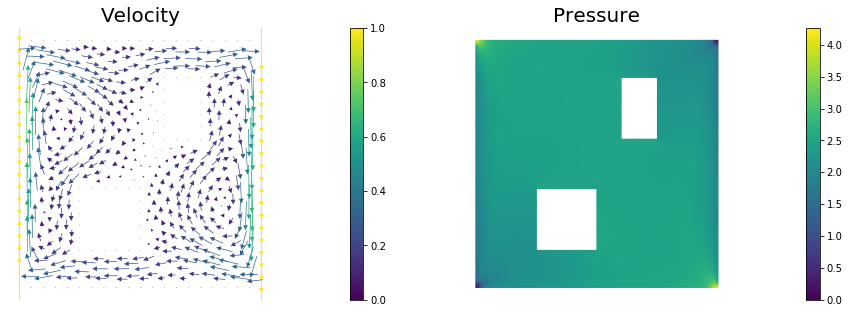Number of dofs: 2023


## 4. Set up model (prior, true/proposed initial condition)

ic_expr = dl.Expression(
'std::min(0.5,std::exp(-100*(std::pow(x-0.35,2) +  std::pow(x-0.7,2))))',
element=Vh.ufl_element())
true_initial_condition = dl.interpolate(ic_expr, Vh).vector()
gamma = 1.
delta = 8.
prior = BiLaplacianPrior(Vh, gamma, delta, robin_bc=True)

prior.mean = dl.interpolate(dl.Constant(0.25), Vh).vector()

t_init         = 0.
t_final        = 4.
t_1            = 1.
dt             = .1
observation_dt = .2

simulation_times = np.arange(t_init, t_final+.5*dt, dt)
observation_times = np.arange(t_1, t_final+.5*dt, observation_dt)

print ("Number of observation points: {0}".format(targets.shape) )
misfit = SpaceTimePointwiseStateObservation(Vh, observation_times, targets)

problem = TimeDependentAD(mesh, [Vh,Vh,Vh], prior, misfit, simulation_times, wind_velocity, True)

objs = [dl.Function(Vh,true_initial_condition),
dl.Function(Vh,prior.mean)]
mytitles = ["True Initial Condition", "Prior mean"]
nb.multi1_plot(objs, mytitles)
plt.show()

Number of observation points: 80## 5. Generate the synthetic observations

rel_noise = 0.01
utrue = problem.generate_vector(STATE)
x = [utrue, true_initial_condition, None]
problem.solveFwd(x[STATE], x)
misfit.observe(x, misfit.d)
MAX = misfit.d.norm("linf", "linf")
noise_std_dev = rel_noise * MAX
parRandom.normal_perturb(noise_std_dev,misfit.d)
misfit.noise_variance = noise_std_dev*noise_std_dev

nb.show_solution(Vh, true_initial_condition, utrue, "Solution")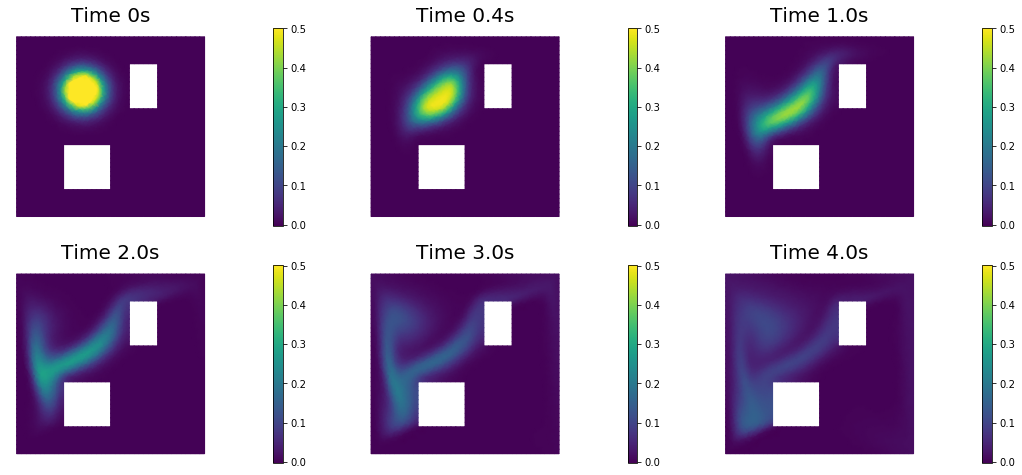## 6. Test the gradient and the Hessian of the cost (negative log posterior)

m0 = true_initial_condition.copy()

(yy, H xx) - (xx, H yy) =  -1.1681934136389683e-13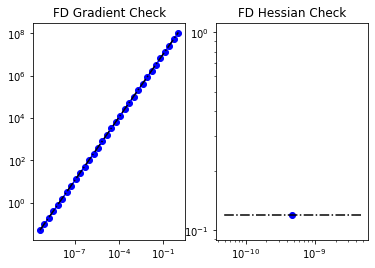[u,m,p] = problem.generate_vector()
problem.solveFwd(u, [u,m,p])
mg = problem.generate_vector(PARAMETER)


(g,g) =  2354159843455358.0


## 8. The Gaussian posterior

H = ReducedHessian(problem, misfit_only=True)

k = 80
p = 20
print( "Single Pass Algorithm. Requested eigenvectors: {0}; Oversampling {1}.".format(k,p) )
Omega = MultiVector(x[PARAMETER], k+p)
parRandom.normal(1., Omega)
lmbda, V = singlePassG(H, prior.R, prior.Rsolver, Omega, k)

posterior = GaussianLRPosterior( prior, lmbda, V )

plt.plot(range(0,k), lmbda, 'b*', range(0,k+1), np.ones(k+1), '-r')
plt.yscale('log')
plt.xlabel('number')
plt.ylabel('eigenvalue')

nb.plot_eigenvectors(Vh, V, mytitle="Eigenvector", which=[0,1,2,5,10,20,30,45,60])

Single Pass Algorithm. Requested eigenvectors: 80; Oversampling 20.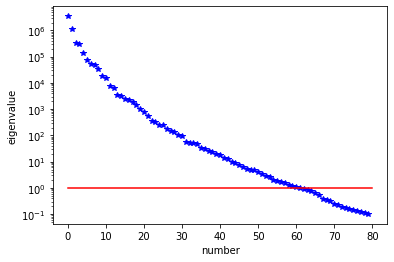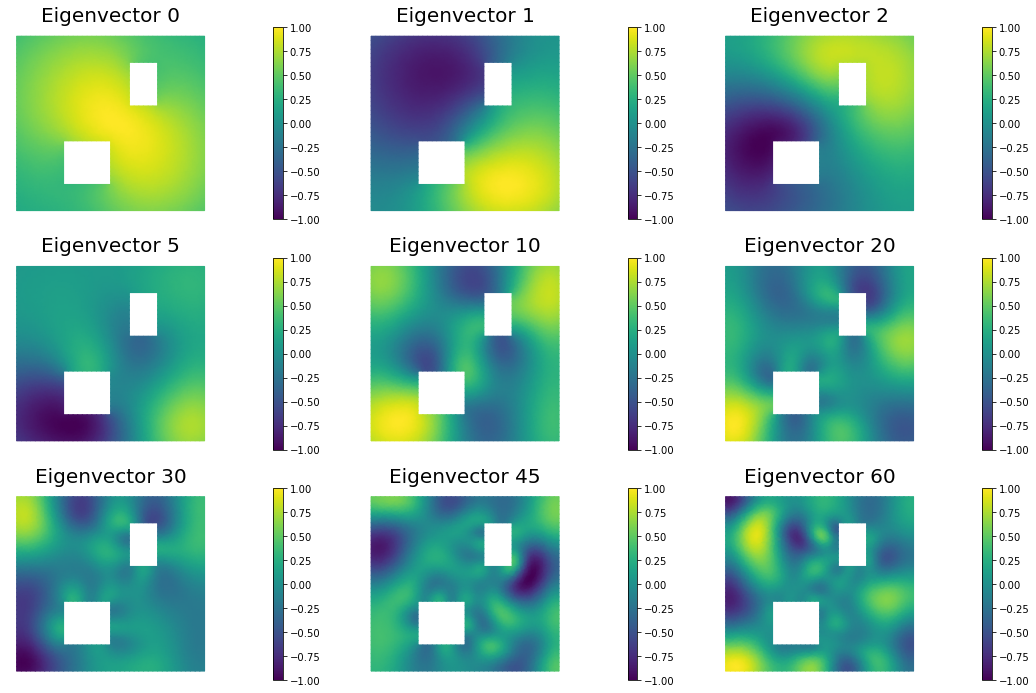## 9. Compute the MAP point

H.misfit_only = False

solver = CGSolverSteihaug()
solver.set_operator(H)
solver.set_preconditioner( posterior.Hlr )
solver.parameters["print_level"] = 1
solver.parameters["rel_tolerance"] = 1e-6
solver.solve(m, -mg)
problem.solveFwd(u, [u,m,p])

total_cost, reg_cost, misfit_cost = problem.cost([u,m,p])
print( "Total cost {0:5g}; Reg Cost {1:5g}; Misfit {2:5g}".format(total_cost, reg_cost, misfit_cost) )

posterior.mean = m

plt.figure(figsize=(7.5,5))
nb.plot(dl.Function(Vh, m), mytitle="Initial Condition")
plt.show()

nb.show_solution(Vh, m, u, "Solution")

 Iterartion :  0  (B r, r) =  1231866.6310868163
Iteration :  1  (B r, r) =  66.31734328582567
Iteration :  2  (B r, r) =  0.3150082598782035
Iteration :  3  (B r, r) =  0.003975346025817018
Iteration :  4  (B r, r) =  1.7003714104952925e-05
Iteration :  5  (B r, r) =  8.577697903362507e-08
Relative/Absolute residual less than tol
Converged in  5  iterations with final norm  0.00029287707153962235
Total cost 782.968; Reg Cost 153.412; Misfit 629.556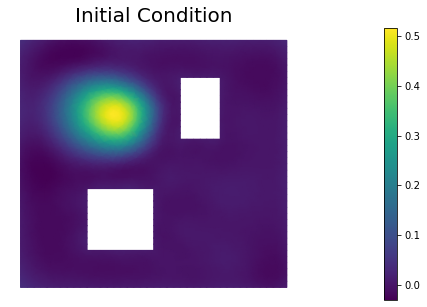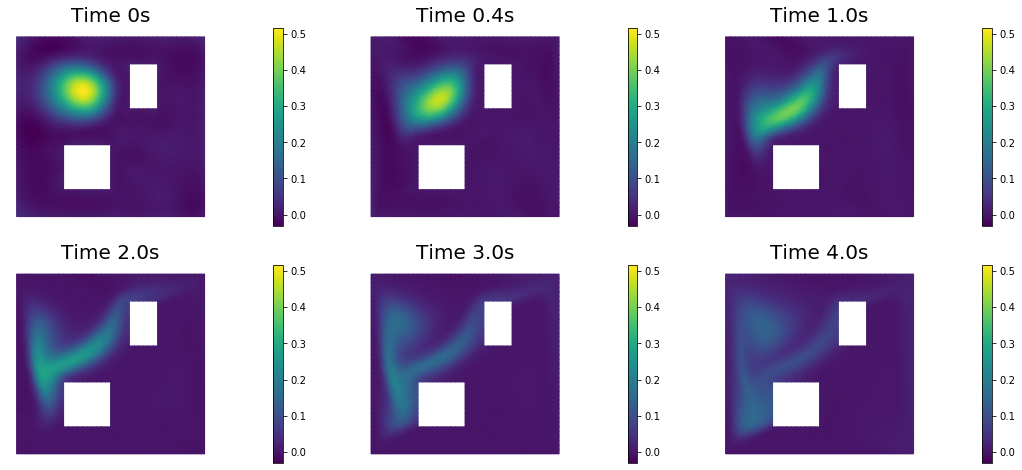## 10. Prior and posterior pointwise variance fields

compute_trace = True
if compute_trace:
post_tr, prior_tr, corr_tr = posterior.trace(method="Randomized", r=300)
print( "Posterior trace {0:5g}; Prior trace {1:5g}; Correction trace {2:5g}".format(post_tr, prior_tr, corr_tr) )
post_pw_variance, pr_pw_variance, corr_pw_variance = posterior.pointwise_variance(method="Randomized", r=300)

objs = [dl.Function(Vh, pr_pw_variance),
dl.Function(Vh, post_pw_variance)]
mytitles = ["Prior Variance", "Posterior Variance"]
nb.multi1_plot(objs, mytitles, logscale=False)
plt.show()

Posterior trace 0.000268048; Prior trace 0.00809706; Correction trace 0.00782902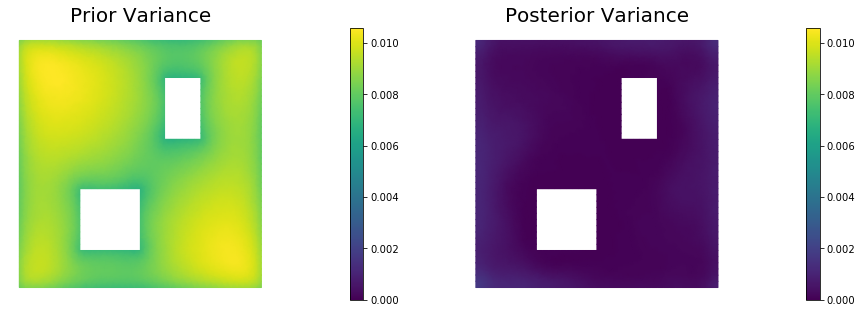## 11. Draw samples from the prior and posterior distributions

nsamples = 5
noise = dl.Vector()
posterior.init_vector(noise,"noise")
s_prior = dl.Function(Vh, name="sample_prior")
s_post = dl.Function(Vh, name="sample_post")

pr_max =  2.5*math.sqrt( pr_pw_variance.max() ) + prior.mean.max()
pr_min = -2.5*math.sqrt( pr_pw_variance.min() ) + prior.mean.min()
ps_max =  2.5*math.sqrt( post_pw_variance.max() ) + posterior.mean.max()
ps_min = -2.5*math.sqrt( post_pw_variance.max() ) + posterior.mean.min()

for i in range(nsamples):
parRandom.normal(1., noise)
posterior.sample(noise, s_prior.vector(), s_post.vector())
plt.figure(figsize=(15,5))
nb.plot(s_prior, subplot_loc=121,mytitle="Prior sample", vmin=pr_min, vmax=pr_max)
nb.plot(s_post, subplot_loc=122,mytitle="Posterior sample", vmin=ps_min, vmax=ps_max)
plt.show()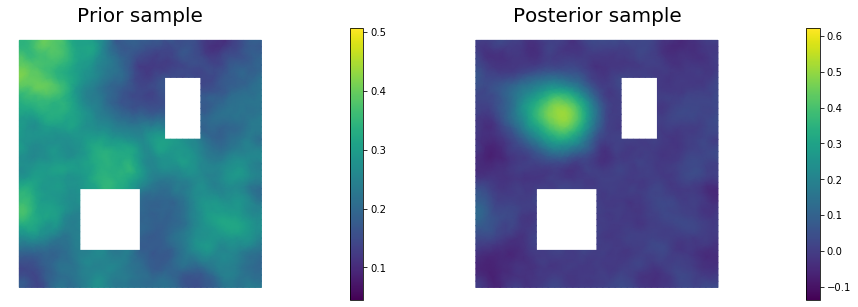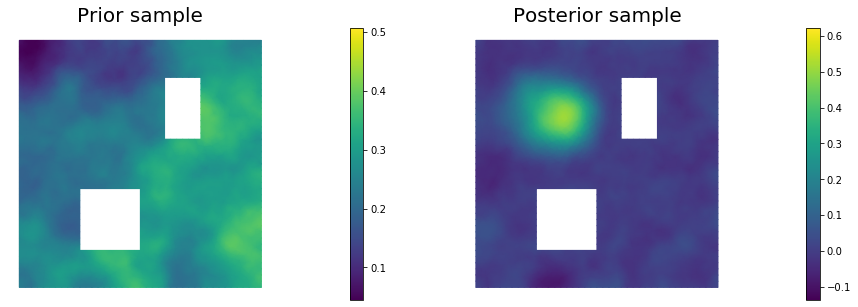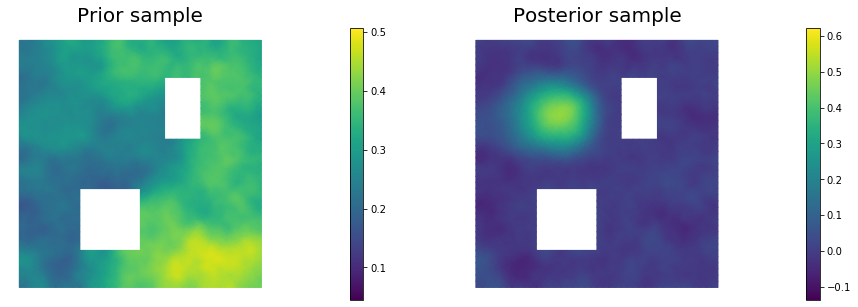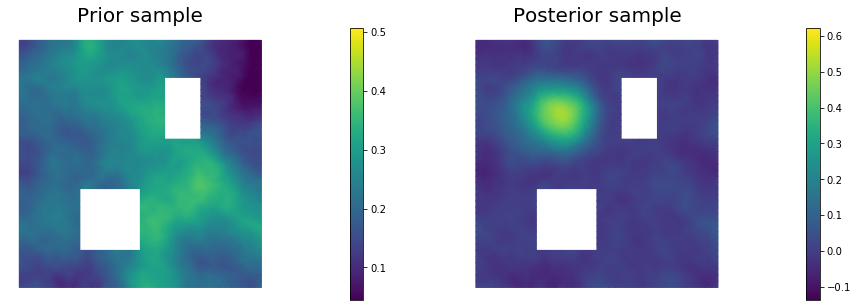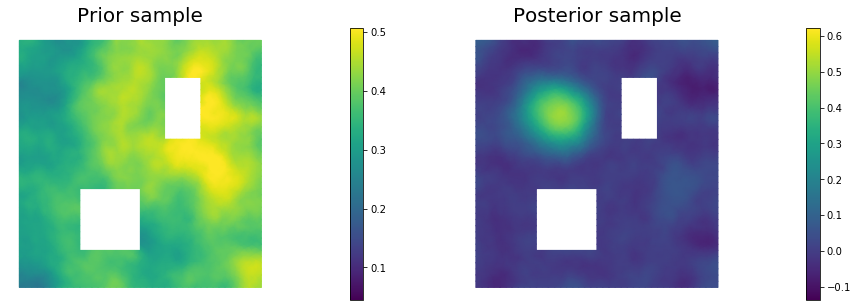Copyright (c) 2016-2018, The University of Texas at Austin & University of California, Merced.
Copyright (c) 2019-2020, The University of Texas at Austin, University of California--Merced, Washington University in St. Louis.